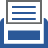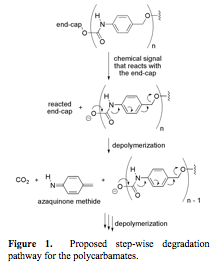##printer friendly Reports: DNI751618-DNI7: Synthesis of Depolymerizable Polymers

Scott Phillips, PhD, Pennsylvania State University

Figure 1.  Proposed step-wise degradation pathway for the polycarbamatesOne of the specific goals of this proposal was to conduct a physical organic study on poly(carbamates) of the general structure shown in Figure 1 to ascertain the effects of structural variations of the repeating units on the rate of analyte-induced depolymerization.  We hypothesized that if we decreased the aromaticity of each repeating unit, then we could decrease the activation energy for depolymerization and accelerate the rate. Similarly, we hypothesized that by increasing the electron density of the aromatic ring in each repeating unit, we could also raise the ground state energy for the starting material, thus decreasing the activation energy required for depolymerization. In this first year of project, we have made substantial progress in testing both hypotheses.

Hypothesis 1: Effect of aromaticity on controlled release in model systems.  Three model compounds (Figure 2) were prepared through relatively short syntheses (Schemes 1 and 2; compound 1 was prepared previously (J. Org. Chem. 2012, 77, 4363)).

Figure 2. Three model compounds used to determine how aromaticity affects the rate of aza-quinone methide formation.  The relative aromaticity values for each ring are obtained from literature values of similar ring systems in the absence of substituents.

Scheme 1. Synthesis of model compound 2a.

Scheme 2. Synthesis of model compound 3a.

These model systems consist of an allyloxycarbonyl (Alloc) group as the reaction based detection unit (which is cleaved selectively in the presence of Pd(0)) and phenol as the signaling molecule. The rate of release of phenol for compounds 1, 2, and 3 was determined by treating each compound with Pd(PPh3)4, Bu3SnH, and acetic acid in THF, then diluting an aliquot of the reaction mixtures in a 1:1 mixture of acetonitrile and pH 7.1 buffered water (Figure 3).  The rate of disappearance of the aniline intermediate was monitored by repetitive injections at 20 min intervals into an LC/MS.

Figure 3. Reaction conditions used for the cleavage of the Alloc group and monitoring the kinetics of phenol release from the three model compounds in Figure 2.

Kinetics of release.  The rates of disappearance of the aniline intermediates are shown as half-lives in Figure 4. The phenanthrene derivative 3 releases phenol the fastest of the three model compounds at a rate of ~51 times faster than the benzene derivative 1.  Naphthalene derivative 2 releases phenol ~12 times faster than compound 1, confirming our hypothesis that a decrease in relative aromaticity increases the rate of aza-quinone methide formation and the subsequent release of a pendant molecule.  Additionally, the "benzylic" C–O bond length was measured via X-ray analysis of crystals of each compound to determine if there is a correlation between the relative aromatic and the bond length.  As seen in previous studies, there appears to be a direct link between this bond length and the relative rate of phenol release.

Figure 4. Effect of aromaticity on the rate of release of phenol.  The half-life values were determined from the experiment described in Figure 3.  The relative aromaticites of ring A are literature values and the C–O bond lengths were determined from X-ray data from crystal structures.

Hypothesis 2: Effect of electron density on depolymerization rates of model oligomers.  The small molecule model systems shown in Figure 5 were designed to evaluate the release time for a single monomer unit from the polymer backbone when the electron density of the repeating unit was modified. Each model system consisted of a reaction-based detection unit, one or two monomer units, and a reporter. An aryl-boronic ester was chosen as the reaction-based detection unit (or end-capping unit) because it reacts selectively under mild conditions to oxidize to a phenol in the presence of hydrogen peroxide.

Figure 5. Four small-molecule model systems. The difference in half-life between the systems containing one monomer unit (A and D) and those containing two monomer units (B and E) gives the half-life for the release of a single monomer unit (C for the H system and F for the OMe system).

The aryl-boronate end-cap was appended to both one and two monomer units. The model systems were terminated with a p-nitroaniline carbamate. Upon degradation of the model systems, the release of p-nitroaniline from the terminal carbamate was monitored using UV/vis spectroscopy. By looking at the difference in the half-lives for release of the p-nitroaniline between the model systems containing one monomer unit and two monomer units, the half-life for the release of a single monomer unit (shown in blue in Figure 5) can be quantified.

The H model systems were synthesized according to the route shown in Scheme 3, and the OMe model systems were prepared as shown in Scheme 4.

Scheme 3. H model systems.a

Scheme 4. OMe model systems.a

Analysis of the model systems to compare the release rates of single monomeric units. The four small molecule model systems shown in Figure 5 depolymerize in the presence of hydrogen peroxide to release p-nitroaniline, which can be detected by UV/vis spectroscopy.  Using this procedure, the half-lives for the H model systems were found to be: 42 min for 12 (one monomer unit) and 634 min for 13 (two monomer units). The difference between the two model systems gives a half-life for a single H monomer of 592 min (Table 1). The half-lives for the OMe model systems were found to be: 11 min for 18 (one monomer unit) and 32 min for 19 (two monomer units). The difference between the two model systems gives a half-life for the OMe monomer of 21 min. This half-life is 28-fold faster than when the OMe group is absent. In a polymeric system, this increase in rate should be compounded to show a dramatic difference in depolymerization times between a polymer comprising the H monomer and one comprising the OMe monomer.

Table 1. Comparison of the half-lives for the release of one monomeric unit.

Concluding remarks. Our goals in the second year of this program are to translate the results of our physical organic studies into highly responsive polymers.

ND
Solid Flow
Instabilities
Dr. Hrenya
UNI
Ionic Liquid
Cage Effects